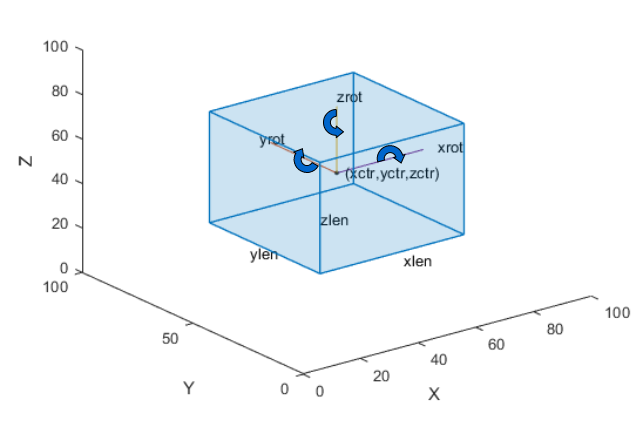# bboxcrop

Crop bounding boxes

## Syntax

``bboxB = bboxcrop(bboxA,window)``
``[bboxB,indices] = bboxcrop(bboxA,window)``
``[___] = bboxcrop(___,Name=Value)``

## Description

example

````bboxB = bboxcrop(bboxA,window)` crops bounding boxes from a set of input bounding boxes, `bboxA`, located in the cropping area, `window`. `bboxB` contains the cropped bounding boxes. This function supports 2-D and 3-D bounding boxes.```
````[bboxB,indices] = bboxcrop(bboxA,window)` additionally returns a vector of indices that indicate which bounding boxes in `bboxA` are within the cropping window, `window`.```
````[___] = bboxcrop(___,Name=Value)` specifies options using one or more name-value arguments in addition to any combination of arguments from previous syntaxes. For example, `OverlapThreshold=1`, sets the positive overlap threshold to `1`.```

## Examples

collapse all

`I = imread('peppers.png');`

Define bounding boxes and labels.

```bboxA = [ 410 230 100 90 186 78 80 60 ]```
```bboxA = 2×4 410 230 100 90 186 78 80 60 ```
```labelsA = [ "garlic" "onion" ];```

Create a center cropping window.

```targetSize = [256 256]; win = centerCropWindow2d(size(I),targetSize);```

Center crop the image.

```[r,c] = deal(win.YLimits(1):win.YLimits(2),win.XLimits(1):win.XLimits(2)); J = I(r,c,:);```

Center crop boxes and labels. Boxes outside the cropping window are removed.

```[bboxB,indices] = bboxcrop(bboxA,win); labelsB = labelsA(indices);```

Display the results.

```figure I = insertObjectAnnotation(I,'Rectangle',bboxA,labelsA); J = insertObjectAnnotation(J,'Rectangle',bboxB,labelsB); imshowpair(I,J,'montage')```## Input Arguments

collapse all

Bounding boxes, specified as an M-by-4, M-by-5, or M-by-9 nonsparse numeric matrix. M is the number of bounding boxes. Each row of the matrix defines a bounding box as either an axis-aligned rectangle, a rotated rectangle, or a cuboid. This table describes the format for each bounding box.

Bounding BoxDescription
Axis-aligned rectangle

Defined in spatial coordinates as an M-by-4 numeric matrix with rows of the form [x y w h], where:

• M is the number of axis-aligned rectangles.

• x and `y` specify the upper-left corner of the rectangle.

• w specifies the width of the rectangle, which is its length along the x-axis.

• h specifies the height of the rectangle, which is its length along the y-axis.

Rotated rectangle

Defined in spatial coordinates as an M-by-5 numeric matrix with rows of the form [xctr yctr xlen ylen yaw], where:

• M is the number of rotated rectangles.

• xctr and yctr specify the center of the rectangle.

• xlen specifies the width of the rectangle, which is its length along the x-axis before rotation.

• ylen specifies the height of the rectangle, which is its length along the y-axis before rotation.

• yaw specifies the rotation angle in degrees. The rotation is clockwise-positive around the center of the bounding box.Cuboid

Defined in spatial coordinates as an M-by-9 numeric matrix with rows of the form [xctr yctr zctr xlen ylen zlen xrot yrot zrot], where:

• M is the number of cuboids.

• xctr, yctr, and zctr specify the center of the cuboid.

• xlen, ylen, and zlen specify the length of the cuboid along the x-axis, y-axis, and z-axis, respectively, before rotation.

• xrot, yrot, and zrot specify the rotation angles of the cuboid around the x-axis, y-axis, and z-axis, respectively. The xrot, yrot, and zrot rotation angles are in degrees about the cuboid center. Each rotation is clockwise-positive with respect to the positive direction of the associated spatial axis. The function computes rotation matrices assuming `ZYX` order Euler angles [xrot yrot zrot].

The figure shows how these values determine the position of a cuboid.Cropping window, specified as a four-element vector, a six-element vector, or a `Cuboid` object. The output bounding box positions are relative to the location of the cropping window.

When you specify `bboxA` as a rectangular input, the cropping window must be a four-element vector in the format [x,y,width,height], or a `Rectangle` object.

When you specify `bboxA` as a cuboid, the cropping window must be a six-element vector in the format [x,y,zwidth,height,depth], or a `Cuboid` object.

### Name-Value Arguments

Specify optional pairs of arguments as `Name1=Value1,...,NameN=ValueN`, where `Name` is the argument name and `Value` is the corresponding value. Name-value arguments must appear after other arguments, but the order of the pairs does not matter.

Before R2021a, use commas to separate each name and value, and enclose `Name` in quotes.

Example: `OverlapThreshold=1`, sets the positive overlap threshold to `1`.

Overlap threshold, specified as a positive scalar less than or equal to `1`. The amount of overlap between the input boxes, `bboxA`, and the cropping area, `window`, is defined as:

`area`(`intersect` (`bboxA`,`window`))/`area`(`union`(`bboxB`,`window`)).

If the computed overlap value is greater than the value of the `threshold` property, then the boxes are clipped to the bounding rectangle border. Otherwise, the boxes are discarded. Lowering the threshold can result in parts of the object getting discarded.

## Output Arguments

collapse all

Cropped bounding boxes, returned as an M2-by-N matrix of M2 bounding boxes. The number of bounding boxes returned is less than the number of bounding boxes in the input. Each row, M2, of the matrix defines one bounding box of the same type as the input `bboxA`. The output bounding box positions are relative to the location of the cropping window.

Indices, returned as a vector of integers. The indices indicate which bounding boxes in the input, `bboxA`, are within the cropping window.

## Version History

Introduced in R2019b

expand all